Question

# Create a step by step mechanism for 1-butanol reacting with acetic acid to form butyl acetate....

Create a step by step mechanism for 1-butanol reacting with acetic acid to form butyl acetate. Under what circumstances would the equilibrium lie to the right? To the left?

We need at least 10 more requests to produce the answer.

0 / 10 have requested this problem solution

The more requests, the faster the answer.

All students who have requested the answer will be notified once they are available.

#### Earn Coins

Coins can be redeemed for fabulous gifts.

Similar Homework Help Questions
• ### Please include labeling of what occurred during each step Physical Data Draw the reaction mechanism of...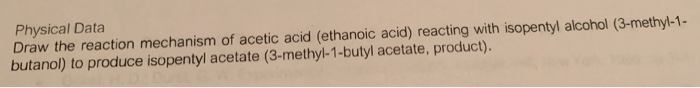Please include labeling of what occurred during each step Physical Data Draw the reaction mechanism of acetic acid (ethanoic acid) reacting with isopentyl alcohol (3-methyl-1- butanol) to produce isopentyl acetate (3-methyl-1-butyl acetate, product).

• ### is my synthesis of butanol from acetic acid done correctly? having issues trying to synthesis butyl...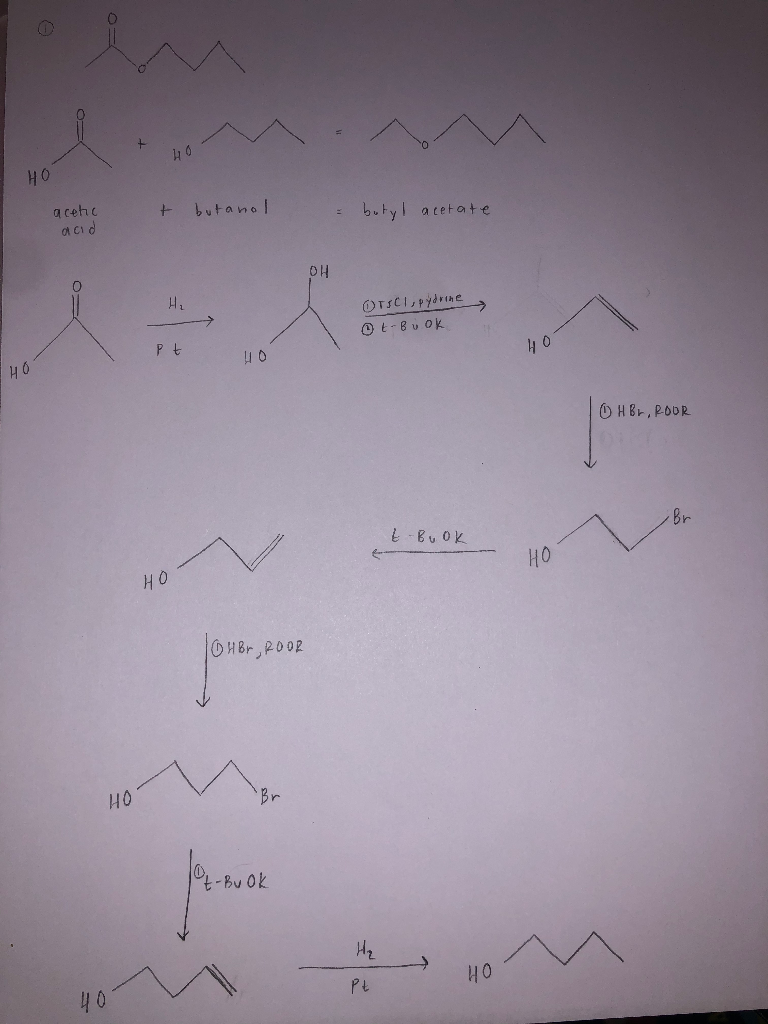is my synthesis of butanol from acetic acid done correctly? having issues trying to synthesis butyl acetate from acetic acid :/ но acetic + butanol = butyl acetate acid ОН OTSCI, pydrine t-Book Pt THO OH Br, ROOR k Bu ok но бHBr 2002 4 - Bu Ok но Ал 10 40

• ### In the experiment of synthesizing isopentyl acetate by reacting an excess of acetic acid with Isopentyl...

In the experiment of synthesizing isopentyl acetate by reacting an excess of acetic acid with Isopentyl alcohol and using sulfuric acid as the catalyst, what was the purpose of doing a distillation after heating under reflux for an hour?

• ### The acetic acid/acetate buffer system is a common buffer used in the laboratory.

The acetic acid/acetate buffer system is a common buffer used in the laboratory. Write the equilibrium equation for the acetic acid/acetate buffer system. The formula of acetic acid is CH3CO2H. equilibrium equation: Which way does the equilibrium shift if more H3O+ is added? The equilibrium will not change. The equilibrium will shift to the left. The equilibrium will shift to the right. Which way does the equilibrium shift if more OH- is added? The equilibrium will shift to the right. The equilibrium will shift to the left. The equilibrium will...

• ### The acetic acid/acetate buffer system is a common buffer used in the laboratory. Write the equilibrium...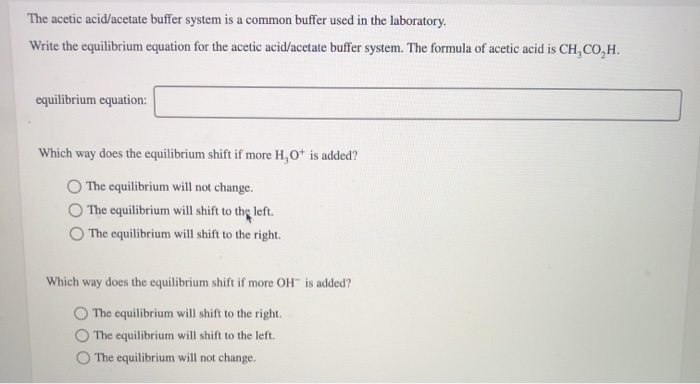The acetic acid/acetate buffer system is a common buffer used in the laboratory. Write the equilibrium equation for the acetic acid/acetate buffer system. The formula of acetic acid is CH,CO,H. equilibrium equation: Which way does the equilibrium shift if more H,O is added? The equilibrium will not change. The equilibrium will shift to the left. The equilibrium will shift to the right. Which way does the equilibrium shift if more OH” is added? The equilibrium will shift to the right...

• ### What is the ratio of undissociated form of acetic acid to acetate at a pH of...

What is the ratio of undissociated form of acetic acid to acetate at a pH of 5.4? The KA of acetic acid is 10-4.7. Determine a.

• ### What concentrations of acetic acid (pKa = 4.76) and acetate would be required to prepare a...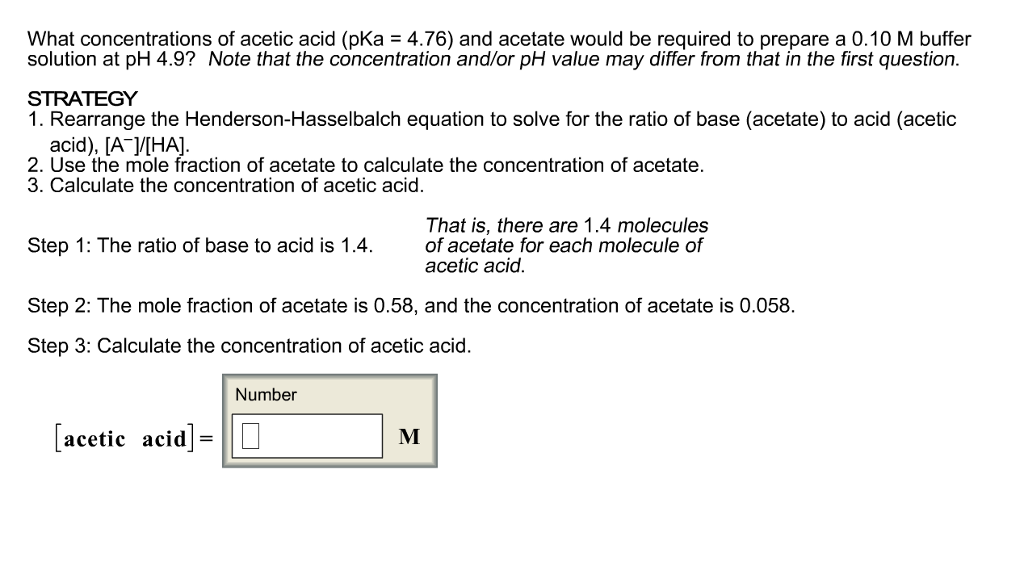What concentrations of acetic acid (pKa = 4.76) and acetate would be required to prepare a 0.10 M buffer solution at pH 4.9? Note that the concentration and/or pH value may differ from that in the first question. 1. Rearrange the Henderson-Hasselbalch equation to solve for the ratio of base (acetate) to acid (acetic acid), [A^-]/[HA]. 2. Use the mole fraction of acetate to calculate the concentration of acetate. 3. Calculate the concentration of acetic acid. That is, there are...

• ### What alcohol IR spectrum is that when reacted with acetic acid UNKNOWN D PPM 112 1-butanol...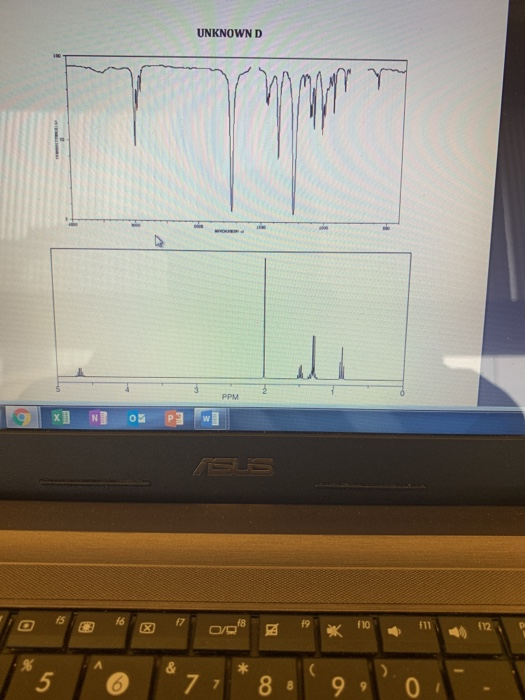What alcohol IR spectrum is that when reacted with acetic acid UNKNOWN D PPM 112 1-butanol (n-butyl alcohol) 2-butanol (sec-butyl alcohol) 2-methyl-1-propanol (isobutyl alcohol) 3-methyl-1-butanol (isopentyl alcohol) 1-pentanol (n-pentyl alcohol) 2-pentanol 3-pentanol cyclopentanol

• ### What concentrations of acetic acid (pKa = 4.76) and acetate would be required to prepare a...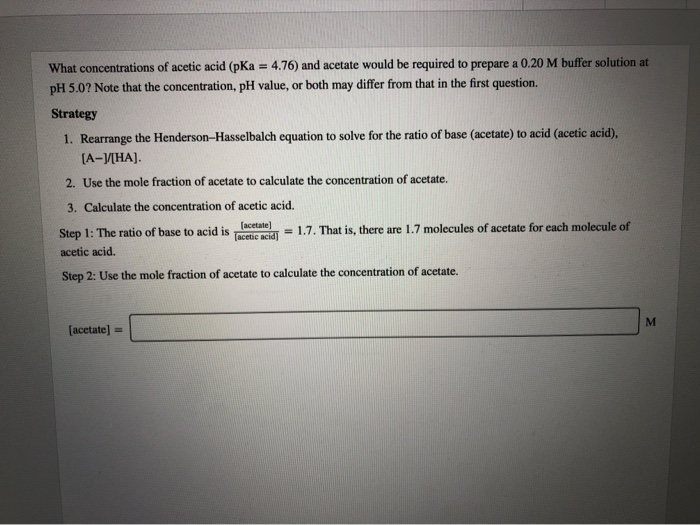What concentrations of acetic acid (pKa = 4.76) and acetate would be required to prepare a 0.20 M buffer solution at pH 5.0? Note that the concentration, pH value, or both may differ from that in the first question. Strategy 1. Rearrange the Henderson-Hasselbalch equation to solve for the ratio of base (acetate) to acid (acetic acid). [A-V[HA). 2. Use the mole fraction of acetate to calculate the concentration of acetate. 3. Calculate the concentration of acetic acid. Step 1:...

• ### ​This experiment involved the use of Fischer Esterification to create isopentyl acetate from reacting isopentyl alcohol...

​This experiment involved the use of Fischer Esterification to create isopentyl acetate from reacting isopentyl alcohol with acetic acid and sufluric acid. 1. Provide a balanced equation to explain the evolution of gas during the sodium bicarbonate washing step. 2. The microscale synthesis of esters often uses a Dean-Stark trap. A Dean-Stark trap separates and collects water during a reaction. How would using a Dean-Stark trap during a Fisher-Esterification affect the synthesis? 3. Esters are often used in artificial flavorings....